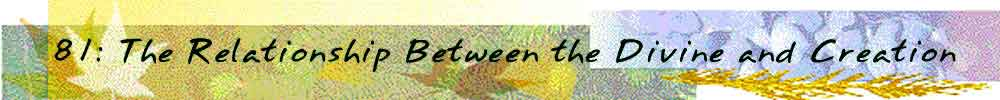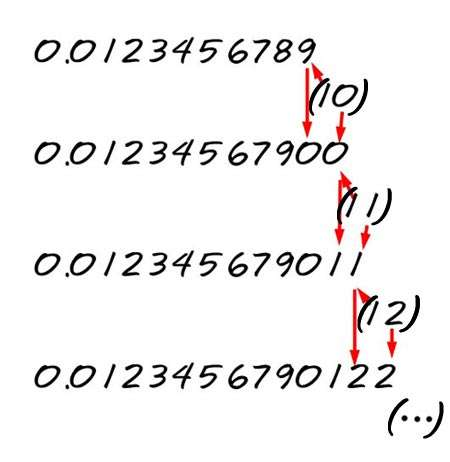Numbers are really interesting. The whole world, even the universe, would not exist without them. Everything is based on numbers, and mathematics. Our entire civilization wouldn't even be as it is without numbers. There is so much you do with them. They show relationships among them, develop along certain paths, like fractals for example, and extend into two, three and even four dimensions, and so on.   Really interesting is the natural (or whole) number 81, and also its relationship to 19, which seem to at the basis of the entire creation of the universe. First I have to explain what a reciprocal is. A reciprocal of a number is written as 1/x, or 1 divided by a number. In the philosophical or spiritual sense, one can see the reciprocal as the relationship of the Divine, represented by the Unity (1), to a number. A few are really special, and can be seen as a mathematical foundation of creation. For Example: the Golden Ratio φ = 1.61803... The Golden Ratio Reciprocal ( Φ ) = 0.61803... Did you notice that the numbers after the dot are the same?  The Golden Ratio Reciprocal ( Φ ) has a unique property by which 1/Φ = Φ + 1; or 1/0.61803... = 1.61803... The Golden Ratio is the only positive number of which its reciprocal is the same number plus one. The Golden Ratio is also called the Golden Section, or Golden Mean. In mathematics, two quantities are in the golden ratio if their ratio is the same as the ratio of their sum to the larger of the two quantities. It is an important ratio that has been used for centuries in in many disciplines, like mathematics, geometry, architecture, etc. It also appears in Nature, and in the Fibonacci sequence in which a number divided by its immediate predecessor in the sequence approaching the Golden Ratio ( 1.6180327868852) with more accuracy as the series continues. You probably have already heard of the Golden Ratio, but does the number 81 ring a bell? Probably not. Yet it is a very special number. In the decimal system the reciprocal of 81, or 1/81 = 0.012345679..., with 012345679 as repeating sequence. It is kind of odd that the number 8 is not present. This the result of the single digit spacing in the decimal system. In other words, every space (after the comma here) can only have one number. But there is another way of writing this out: we place a number on every spacing without reducing it to a single digit. Every spacing is represented by the accolades: (0),(1)(2)(3)(4)(5)(6)(7)(8)(9)(10)(11)(12)(13)(14)... We will show here that this way of writing is the same as the decimal system. It is the same number. If I were to write this number allotting only one digit space per next appearing number in order to write it in the decimal system), I have to make adjustments. According to the rule of addition, the 10 that follows the 9 forces this 9 to become a 10, of which I can only write the zero, and forces the 8 before it into a 9, while the 7 before that is not affected.This is how we add numbers in the decimal system. So, for the 10, we have to write down 0, and add 1 to the next digit to the left. 1 added to the 9 is 0 and we have to add a 1 the its left to, what changes the 8 to 9. We do the same for every other number after that, 11, 12, 13 ..., which gives us eventually the decimal notation of 0.012345679012345679.... In other words, 0.012345679... is the same as all the natural numbers lined up, one next to one another. That is ALL the natural numbers in the universe into infinity. Thus, the reciprocal of All the natural numbers in the universe is 81. One could say the Divine, or the Unity relates to all the natural numbers by the number 81. Thus, 81 is a special number. 81 = 9 x 9 = 9² or 3⁴; and 8+1=9 Nine has always been regarded as a number of completion, of totality. The decimal system has a total of nine numbers. 1 is the unity or beginning, 9 is the end or completion. With a Greek expression: 1 is the Alpha and 9 is the Omega. 1 and 9 = 19; 19+81=100, a unity of a higher order. When we make the reciprocal of 19, or 1/19 = 0,052631578947368421...., then 052631578947368421 is the repeating sequence. When we add up all the numbers in this repeating sequence, or 0 + 5 + 2 + 6 + 3 + 1 + 5 + 7 + 8 + 9 + 4 + 7 + 3 + 6 + 8 + 4 + 2 + 1 = 81! Worth while to mention is that the 5 Platonic Solids plus the 13 Archimedian Solids plus the sphere in which they all can be enclosed (all vertexes or corners must touch the sphere) makes 19 in total.